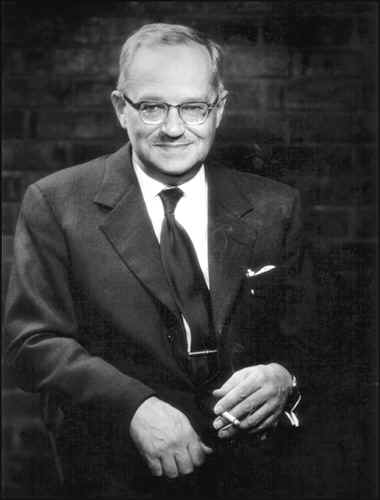Fisher 的随机化检验，针对的是如下的零假设，又被称为 sharp null：  $$H_0 : Y_i(1) = Y_i(0), \forall i = 1,\cdots,n.$$ 坦白地说，这个零假设是我见过的最奇怪的零假设，没有之一。现行的统计教科书中，讲到假设检验，零假设都是针对某些参数的，而 Fisher 的 sharp null 看起来却像是针对随机变量的。这里需要讲明白的是，当我们关心**有限样本 （finite sample）**的因果作用时，每个个体的潜在结果 $\{Y_i(1), Y_i(0)\}$ 都是固定的，观测变量 $Y_i = Z_i Y_i(1) + (1 – Z_i)Y_i(0)$ 的随机性仅仅由于“随机化” $Z_i$ 本身导致的。理解清楚这点，才能理解 Fisher randomization test 和后面的 Neyman repeated sampling procedure。如果读者对于这种有限样本的思考方式不习惯，可以先阅读一下经典的抽样调查教科书，那里几乎全是有限样本的理论，所有的随机性都来自于随机采样的过程。

$$P( \overrightarrow{Z} | \overrightarrow{Y} ) = \binom{n}{m}^{-1}, \forall \overrightarrow{Y} ,$$来检验零假设，问题在于这个统计量的分布不易求出。但是，我们又知道，这个统计量的分布完全来自随机化。因此，我们可以用如下的“随机化”方法 （Monte Carlo 方法模拟统计量的分布）：将处理分配机制的向量 $\overrightarrow{Z}$ 进行随机置换得到$\overrightarrow{Z}^1 = (Z_1^1, \cdots, Z_n^1)$，计算此时的检验统计量 $T^1 = T(\overrightarrow{Z}^1, \overrightarrow{Y})$；如此重复多次（$n$ 不大时，可以穷尽所有的置换），便可以模拟出统计量在零假设下的分布，计算出 p 值。

$\hat{\text{Var}}(\hat{\tau}) = \sum\limits_{Z_i=1} (Y_i – \bar{y}_1)^2 /(m-1)m+ \sum\limits_{Z_i=0} (Y_i – \bar{y}_0)^2/(n-m-1)(n-m)$

1. 在 sharp null下，Neyman 方法下构造的 T 统计量，是否和 Fisher randomization test 构造的统计量相同？分布是否相同？
2. Fisher randomization test 中的统计量可以有其他选择，比如 Wilcoxon 秩和统计量等，推断的方法类似。
3. $Y$ 是二值变量时，上面 Fisher 的方法就是教科书中的 Fisher exact test。在没有学习 potential outcome 这套语言之前，理解 Fisher exact test 是有些困难的。
4. 证明 $E \{ \hat{\text{Var}}(\hat{\tau}) \} \geq \text{Var}(\hat{\tau})$
5. 假定 $n$ 个个体是一个超总体（super-population）的随机样本，超总体的平均因果作用定义为 $$\tau_{SP} = E\{ Y(1) – Y(0) \}.$$ 那么 Neyman 的方法得到估计量是超总体平均因果作用的无偏估计，且方差的表达式是精确的；而 sharp null 在超总体的情形下不太适合。

• Neyman, J. (1923) On the application of probability theory to agricultural experiments. Essay on principles. Section 9. reprint in Statistical Science. 5, 465-472. with discussion by Donald Rubin.